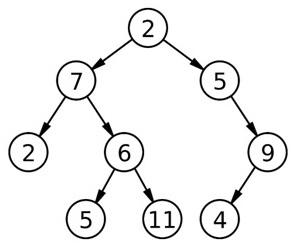# Print Boundary Sum of a Binary Tree

In-this article, we are going to learn how to find boundary sum of a binary tree? This article contains the solution along with algorithm and program.
Submitted by Radib Kar, on December 02, 2018

Problem statement: Given a binary tree, print the boundary sum of the tree.

Solution:

First of all we need to understand what the boundary sum of a binary tree is? It's simply the cumulative sum of all boundary nodes surrounding the tree. For the following example:The boundary nodes are: 2, 7, 2, 5, 11, 4, 9, and 5 (from left to right direction)

So there are four types of node considered to be boundary node:

1. Root node
2. All the leaf nodes
3. All the nodes at the leftmost side (there will be a duplicate since the last leftmost node is itself a leaf node, discard the duplicate)
4. All the nodes at the rightmost side ( there will be a duplicate since the last rightmost node is itself a leaf node, discard the duplicate)

Thus, the boundary sum is: 45

Algorithm:

1. Set sum=0
2. Add root->data to sum; (type-1 boundary node)
3. Find all the leaf nodes & add their values respectively. (type-2 boundary node)
For this portion we do a level-order traversal & keep checking whether the traversed node has both its left & right point NULL or not.
If the traversed node has both its pointer NULL then it’s a leaf node & of course add to sum cumulatively.
4. Find the leftmost nodes (without leaf node) & add their respective values. (type-3 boundary node)
Set temp to root->left
5. ```while(!(temp->right==NULL&&temp->left==NULL)){//avoiding leaf node
//always tend to move left side
//first for left most nodes
if(temp->left)
temp=temp->left;
else
temp=temp->right;
}
```
6. Find the rightmost nodes (without leaf node) & add their respective values. (type-4 boundary node)
Set temp to root->right
7. ```while(!(temp->right==NULL&& temp->left==NULL)){//avoiding leaf node
//always tend to move right side
//first for right most nodes
if(temp->right)
temp=temp->right;
else
temp=temp->left;
}
```

## C++ program to print Boundary Sum of a Binary Tree

```#include <bits/stdc++.h>
using namespace std;

// tree node is defined
class tree{
public:
int data;
tree *left;
tree *right;
};

int findBoundarySum(tree* root){ //finding boundary sum
if(root==NULL) //base case
return 0;
int sum=0; //(step1)
sum+=root->data; //add root value (step2)

//find the leaf nodes & add(step3)
tree* temp=root, *store; //copy root to temp
queue<tree*> q; //creat a queue to store tree* variables (pointer to nodes)

//doing level order traversal
q.push(temp);
while(!q.empty()){
store=q.front();
q.pop();
// if left & right both pointers are NULL, it's a leaf node
if(store->left==NULL && store->right==NULL)
sum+=store->data; // add leaf node value
if(store->left)
q.push(store->left);
if(store->right)
q.push(store->right);
}

/////end of step3////////

//adding the leftmost nodes excluding leaf node(step4)///////////
temp=root->left;
while(!(temp->right==NULL && temp->left==NULL)){//avoiding leaf node
sum+=temp->data;
if(temp->left)
temp=temp->left;
else
temp=temp->right;
}

////end of step4//////////

//adding the rightmost nodes excluding leaf node(steps)///////////
temp=root->right;
while(!(temp->right==NULL && temp->left==NULL)){//avoiding leaf node
sum+=temp->data;
if(temp->right)
temp=temp->right;
else
temp=temp->left;
}
////end of step5//////////
//boundary sum is now calculated, return it
return sum;
}

// creating new node
tree* newnode(int data)
{
tree* node = (tree*)malloc(sizeof(tree));
node->data = data;
node->left = NULL;
node->right = NULL;
return(node);
}

int main()
{
//**same tree is builted as shown in example**
cout<<"Tree is built like the example aforesaid"<<endl;
//building the tree like as in the example
tree *root=newnode(2);
root->left= newnode(7);
root->right= newnode(5);
root->right->right=newnode(9);
root->right->right->left=newnode(4);
root->left->left=newnode(2);
root->left->right=newnode(6);
root->left->right->left=newnode(5);
root->left->right->right=newnode(11);

cout<<"finding boundary sum......"<<endl;
cout<<"boundary sum is "<<findBoundarySum(root)<<endl;

return 0;
}
```

Output

```Tree is built like the example aforesaid
finding boundary sum......
boundary sum is 45
```

What's New (MCQs)

Top Interview Coding Problems/Challenges!

Languages: » C » C++ » C++ STL » Java » Data Structure » C#.Net » Android » Kotlin » SQL
Web Technologies: » PHP » Python » JavaScript » CSS » Ajax » Node.js » Web programming/HTML
Solved programs: » C » C++ » DS » Java » C#
Aptitude que. & ans.: » C » C++ » Java » DBMS
Interview que. & ans.: » C » Embedded C » Java » SEO » HR
CS Subjects: » CS Basics » O.S. » Networks » DBMS » Embedded Systems » Cloud Computing
» Machine learning » CS Organizations » Linux » DOS
More: » Articles » Puzzles » News/Updates

© https://www.includehelp.com some rights reserved.